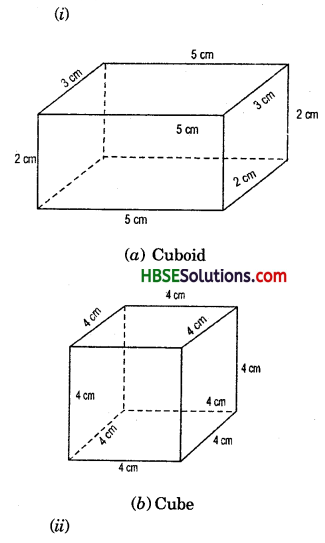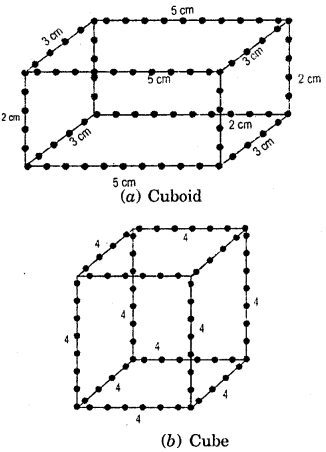# HBSE 7th Class Maths Solutions Chapter 15 Visualising Solid Shapes Ex 15.2

Haryana State Board HBSE 7th Class Maths Solutions Chapter 15 Visualising Solid Shapes Ex 15.2 Textbook Exercise Questions and Answers.

## Haryana Board 7th Class Maths Solutions Chapter 15 Visualising Solid Shapes Exercise 15.2

Question 1.
Use isometric dot paper and make an isometric sketch for each one of the given shapes:
Solution:
Let us take m to be the number of pannit’s marbles.Fig. 15.16.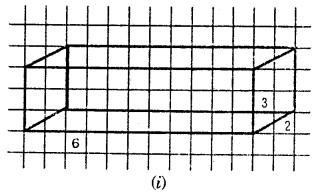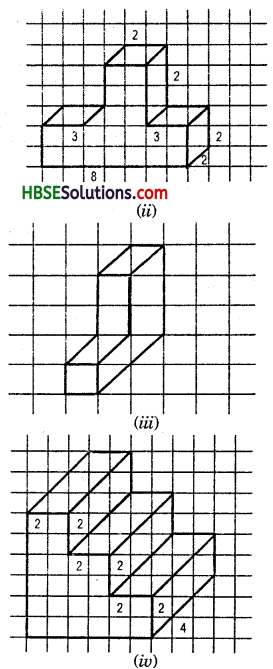Question 2.
The dimensions of a cuboid are 5 cm, 3 cm and 2 cm. Draw three different isometric sketches of this cuboid.
Solution: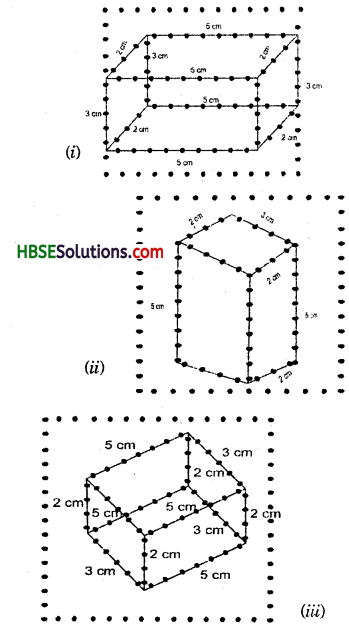Question 3.
Three cubes each with 2 cm edge are placed side by side to form a cuboid. Sketch an oblique or isometric sketch of this cuboid.
Solution: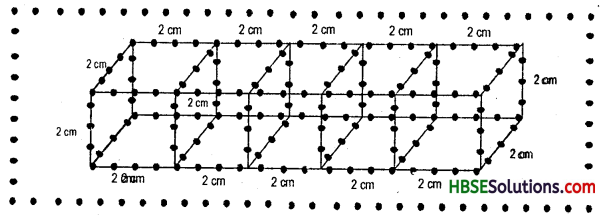Question 4.
Make an oblique sketch for each one of the given isometric shpaes:
Solution: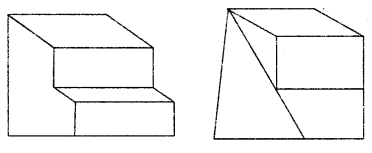Question 5.
Give (i) an oblique sketch and (ii) an isometric sketch for each of the following :
(a) A cuboid of dimensions 5 cm, 3 cm and 2 cm. (Is your sketch unique ?)
(b) A cube with an edge 4 cm long.
An Isometric sheet is attached at the end of the book. You could try to make on it some cubes or cuboids of dimensions specified by your friend.
Solution: